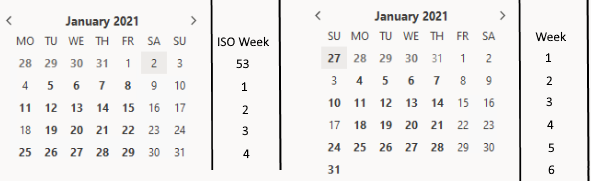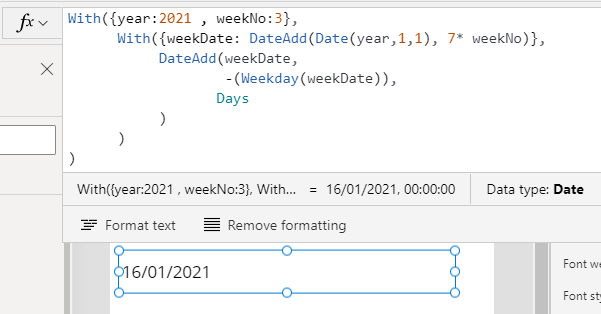# Dates - How to get the start date from week number

When working with dates, there may be the requirement to derrive the start date of a week based on a week number. This post describes the formula to carry out this task.

There may be a requirement to get the start date of a week based on a week number. For example, given the input value 'week 3 /2021', the requirement is to return Monday 18th January 2021.

It's relatively simple to carry out this task - we can add the required number of weeks to the 1st January for the target year, and we can then work out the Monday (or start date of the week) using the formula that I describe here:

However, this task may not be as simple as we imagine because there are different standards for numbering weeks.

## What's the difference between Week Numbers and ISO Week Numbers?

As I describe in my post here, we can use the ISO or non ISO methods to number weeks.

To summarise this post, the image beneath illustrates how according to the ISO scheme, January 1st 2021 is week 53 whereas using the standard week numbering scheme, January 1st will always be week 1.## 1 - How to get the start date of the week from a standard week number

Taking the simpler example of how to get the start date of a week from a standard week number, we can use the formula beneath. We would replace the year and weekNo values with our target values.

`With({year:2021 , weekNo:3},      With({weekDate: DateAdd(Date(year,1,1), 7* weekNo)},           DateAdd(weekDate,                   -(Weekday(weekDate)),                  Days           )      ))`
With standard week numbers, we add the target number of weeks to the 1st January of the target year. Note that the DateAdd function doesn't include the ability to add weeks to an input value. For this reason, we multiply the target week value by 7 and add the output as days.

As the screenshot beneath shows, this formula returns our expected value - Sunday 10th January 2021.## 2 - How to get the start date of the week from an ISO week number

It's more difficult to retrieve the start date of a week based on an ISO week number because January 1st may not be week 1, as is the case with 2021. In these cases, we can call an If statement to offset the result by 1 week when the ISOWeekNum value of January 1st doesn't equal 1. Here's the formula we would use.

`With({year:2021 , weekNo:35},With({weekDate:      If(ISOWeekNum(Date(year,1,1)) < 1,         DateAdd(Date(year,1,1), 7* (weekNo-1)),         DateAdd(Date(year,1,1), 7* weekNo)      )      },      DateAdd(weekDate,        -(Weekday(weekDate,StartOfWeek.MondayZero)),        Days   ) ))`
The other notable thing is that with ISO week numbers, the start of the week occurs on Monday. The Weekday function caters mainly for US users where the start of the week begins on Sunday, therefore, the part of the formula that retrieves the start of the week must specify Monday as the start of the week.

As the screenshot beneath shows, this formula returns our expected value - Monday 18th January 2021.## Conclusion

To get the start date of a week based on a week number, we can add the required number of weeks to the 1st January for the target year, and we can then work the start date of the week. If the input value is an ISO week number, we may need to offset the result by 1 week if the 1st January is week 53.